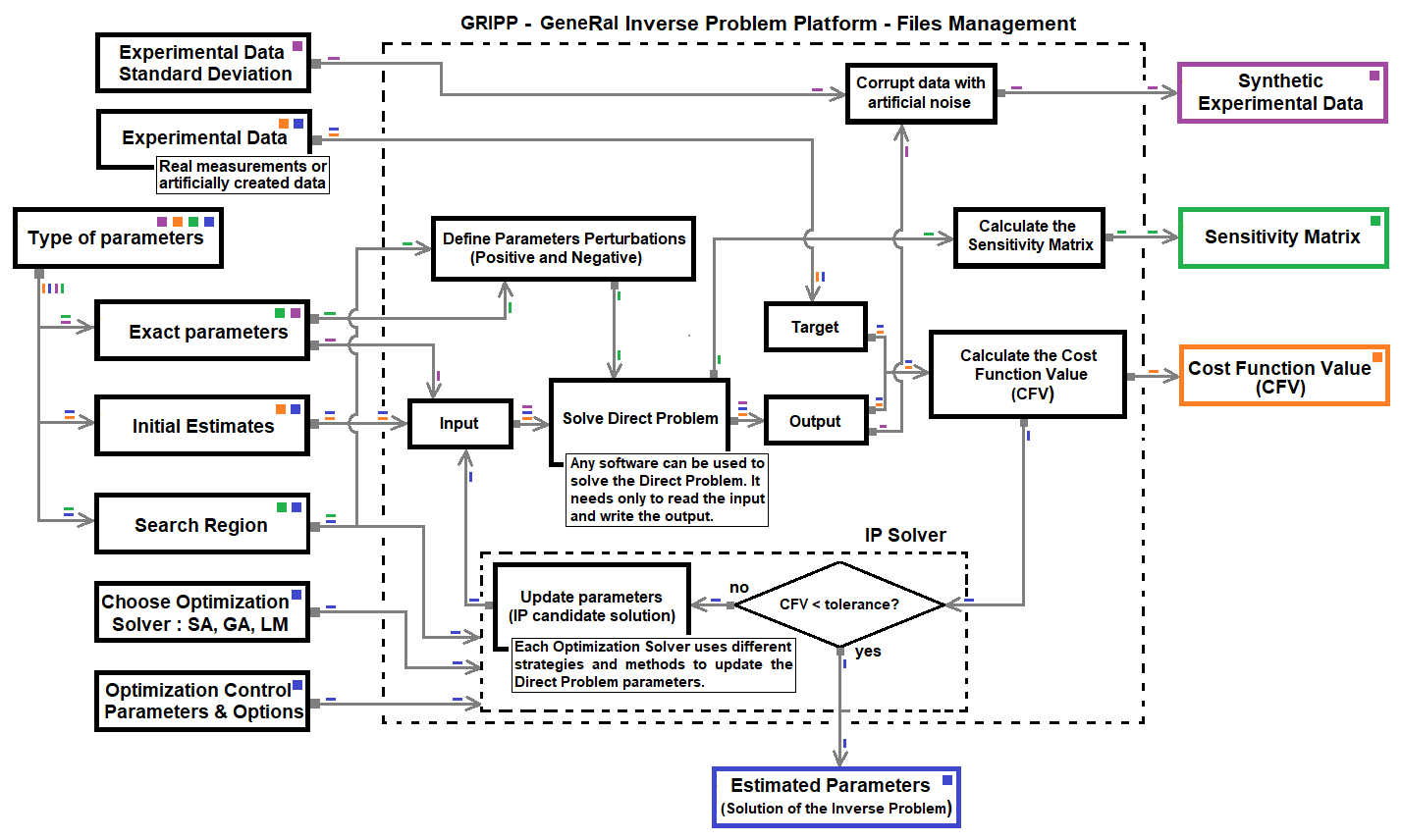# gripp_details

The gripp package [@R-gripp] can be described as a set of functions designed to solve inverse problems.

The use of different Inverse Problems solvers and sensitivity analysis is a research topic of interest in many fields, such as drying simulation , polution dispersion in rivers , and adsorption simulation .

The direct problem is used to calculate a cost function to be minimized. The inverse problem is solved using an external package function chosen by the user: GA; GenSA; and others solvers, yet to be implemented.

This document is an introduction to the gripp package, where it will offer some examples to be used to adapt the package to your specific problem. The first step is to install the package into your section using:

library(gripp)

## Examples

The gripp Help Pages for each function (cost, invprob, sensitivity and synthetic) describe a simple example for them that can be used. In all Help Pages, the examples consider that the direct problem is solved using a function implemented in the R platform. The gripp is also able to run an external software, changing an input file when needed and saving the results obtained during the process. Fig. 1 is a schematic representation of the gripp package, with the information needed for each function and their product.

It is important to say that the examples suplied in gripp Help Pages are made to work quickly and to be tested inside the CRAN Platform, therefore they do not save variables ou results on disk. That characteristic is configured setting up “isitR” value to TRUE. When you are using some different software to solve the Direct Problem, you must set “isitR” to FALSE, as well as, configure the information about the files that are needed (input, output and the command).

All functions will need some information about the direct problem, the target, or even the experimental standard deviation of the error. Each specific function Help Page contains a variation of the same schematic representation of the gripp package, including detailed information for each one, that is, cost, invprob, sensibility, and synthetic.Fig. 1 - General Inverse Problem Platform

1) cost The cost function can be used to calculate the squared difference between the target value and direct problem solution using some specified parameter.

2) invprob The invprob function is used to solve the inverse problem. The user can choose among different solvers and can setup some control parameters. The result of this function will be a list: a value with the quadratic residue calculated; the parameters estimated; and the number of function call needed to solve the inverse problem.

3) sensitivity The sensitivity function can calculate the sensitivity matrix, that is, the first order derivative of the direct problem for each considered parameter. The derivatives are calculated using a central difference scheeme. The sensitivity matrix can help the user to decide which parameters can be estimated or not.

4) synthetic The Direct Problem will be solved and the result will be corrupted to simulate experimental error. The user can inform the error Standard Deviation that will be added to the solution. The function will return data obtained using the equation:

Simulated_Results_with_Error <- Direct_Problem_Results * (1 + sigma).